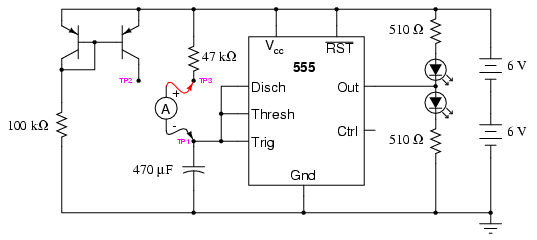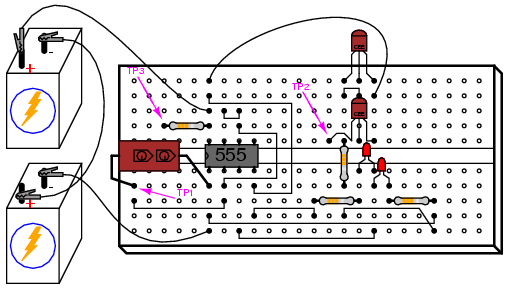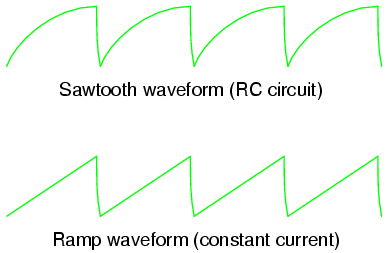» Experiment » Calculator/Converters » Radio » Newsletter » Associations and Societies » Component Manufacturers

 Electronics Symentics A B C D E F G H I J K L M N O P Q R S T U V W X Y Z

## 555 ramp generator

PARTS AND MATERIALS

• Two 6 volt batteries

• One capacitor, 470 µF electrolytic, 35 WVDC (Radio Shack catalog # 272-1030 or equivalent)

• One capacitor, 0.1 µF, non-polarized (Radio Shack catalog # 272-135)

• One 555 timer IC (Radio Shack catalog # 276-1723)

• Two PNP transistors -- models 2N2907 or 2N3906 recommended (Radio Shack catalog # 276-1604 is a package of fifteen PNP transistors ideal for this and other experiments)

• Two light-emitting diodes (Radio Shack catalog # 276-026 or equivalent)

• One 100 kΩ resistor

• One 47 kΩ resistor

• Two 510 Ω resistors

The voltage rating on the 470 µF capacitor is not critical, so long as it generously exceeds the maximum power supply voltage. In this particular circuit, that maximum voltage is 12 volts. Be sure you connect this capacitor in the circuit properly, respecting polarity!

CROSS-REFERENCES

Lessons In Electric Circuits, Volume 1, chapter 13: "Capacitors"

Lessons In Electric Circuits, Volume 4, chapter 10: "Multivibrators"

LEARNING OBJECTIVES

• How to use the 555 timer as an astable multivibrator

• A practical use for a current mirror circuit

• Understanding the relationship between capacitor current and capacitor voltage rate-of-change

SCHEMATIC DIAGRAMILLUSTRATIONINSTRUCTIONS

Again, we are using a 555 timer IC as an astable multivibrator, or oscillator. This time, however, we will compare its operation in two different capacitor-charging modes: traditional RC and constant-current.

Connecting test point #1 (TP1) to test point #3 (TP3) using a jumper wire. This allows the capacitor to charge through a 47 kΩ resistor. When the capacitor has reached 2/3 supply voltage, the 555 timer switches to "discharge" mode and discharges the capacitor to a level of 1/3 supply voltage almost immediately. The charging cycle begins again at this point. Measure voltage directly across the capacitor with a voltmeter (a digital voltmeter is preferred), and note the rate of capacitor charging over time. It should rise quickly at first, then taper off as it builds up to 2/3 supply voltage, just as you would expect from an RC charging circuit.

Remove the jumper wire from TP3, and re-connect it to TP2. This allows the capacitor to be charged through the controlled-current leg of a current mirror circuit formed by the two PNP transistors. Measure voltage directly across the capacitor again, noting the difference in charging rate over time as compared to the last circuit configuration.

By connecting TP1 to TP2, the capacitor receives a nearly constant charging current. Constant capacitor charging current yields a voltage curve that is linear, as described by the equation i = C(de/dt). If the capacitor's current is constant, so will be its rate-of-change of voltage over time. The result is a "ramp" waveform rather than a "sawtooth" waveform:The capacitor's charging current may be directly measured by substituting an ammeter in place of the jumper wire. The ammeter will need to be set to measure a current in the range of hundreds of microamps (tenths of a milliamp). Connected between TP1 and TP3, you should see a current that starts at a relatively high value at the beginning of the charging cycle, and tapers off toward the end. Connected between TP1 and TP2, however, the current will be much more stable.

It is an interesting experiment at this point to change the temperature of either current mirror transistor by touching it with your finger. As the transistor warms, it will conduct more collector current for the same base-emitter voltage. If the controlling transistor (the one connected to the 100 kΩ resistor) is touched, the current decreases. If the controlled transistor is touched, the current increases. For the most stable current mirror operation, the two transistors should be cemented together so that their temperatures never differ by any substantial amount.

This circuit works just as well at high frequencies as it does at low frequencies. Replace the 470 µF capacitor with a 0.1 µF capacitor, and use an audio detector to sense the voltage waveform at the 555's output terminal. The detector should produce an audio tone that is easy to hear. The capacitor's voltage will now be changing much too fast to view with a voltmeter in the DC mode, but we can still measure capacitor current with an ammeter.

With the ammeter connected between TP1 and TP3 (RC mode), measure both DC microamps and AC microamps. Record these current figures on paper. Now, connect the ammeter between TP1 and TP2 (constant-current mode). Measure both DC microamps and AC microamps, noting any differences in current readings between this circuit configuration and the last one. Measuring AC current in addition to DC current is an easy way to determine which circuit configuration gives the most stable charging current. If the current mirror circuit were perfect -- the capacitor charging current absolutely constant -- there would be zero AC current measured by the meter.